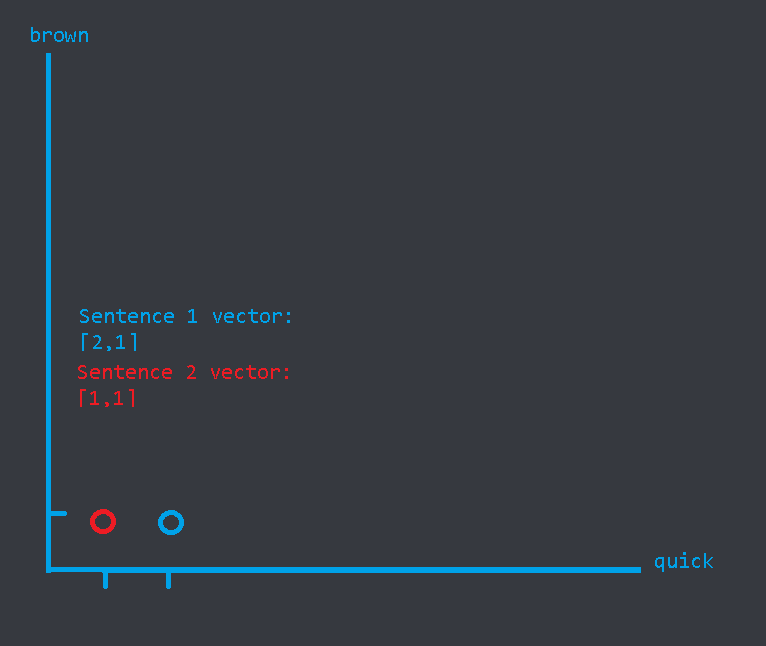Introduction to cosine similarity

In this tutorial, I will try and take you through what cosine similarity is and how it works, as well as the code.

The full code and how to use it:

``````import nltk
import string
from sklearn.feature_extraction.text import TfidfVectorizer

# pickle
stemmer = nltk.stem.porter.PorterStemmer()
remove_punctuation_map = dict((ord(char), None) for char in string.punctuation)

def stem_tokens(tokens):
return [stemmer.stem(item) for item in tokens]

def normalize(text):
return stem_tokens(nltk.word_tokenize(text.lower().translate(remove_punctuation_map)))

vectorizer = TfidfVectorizer(tokenizer=normalize, stop_words='english')

def similarity(text1, text2):
tfidf = vectorizer.fit_transform([text1, text2])
return ((tfidf * tfidf.T).A)[0, 1]``````

To use it, simply run the similarity function using the two texts that you would like to compare as parameters.
This is incredibly useful for search within your code, or if you would like to make a fast-running chatbot system. I used this algorithm for many of my projects as well.

### How it works

Let's find the cosine similarity mathematically (i.e. without a computer) for two different sentences:
Sentence 1 is "The quick quick brown fox jumps over the dog"
Sentence 2 is "The quick brown spider crawls over the lazy bug"

The first and foremost thing our engine does is remove stop words.
These are words that don't necessarily change the theme or meaning of a sentence but need to be there for grammar's sake. If you want to know what they are, this gist may be helpful https://gist.github.com/sebleier/554280.

After removing stop words, our sentences look like this:
Sentence 1 is "quick quick brown fox jumps dog"
Sentence 2 is "quick brown spider crawls lazy bug"

The next thing to do now is to plot this on a vector space.
In this space, each axis represents one of the common words between the sentences. In this case, the number of common non-stop words is two (making this a relatively simple calculation for ease of understanding).

The number on the axis represents the word count of each of the common words.
This is what the vector space looks like:We then find the vectors of each of the sentences ([2,1] and [1,1] respectively) and move on to the next step which is substituting these into the cosine similarity formula which looks like this:The first step to do is find the dot product of the two vectors, i.e. the A ⋅ B.
This is simple with our vectors [2,1] and [1,1].
The dot product is `2*1 + 1*1` which is equal to 3.
Then the second part is dividing this by the root of the sum of the square of each value in the first vector multiplied by all this again for the second vector.

The value of this for vector A (sentence 1) is the square root of 2^2 + 1^2 which is the square root of 5. This is then multiplied by the value for vector B (sentence 2) which is the square root of 1^2 + 1^2 which is the square root of 2.

So the final similarity is 3/sqrt(5)*sqrt(2).
Thanks for reading this tutorial! An upvote will be much appreciated.

You are viewing a single comment. View All